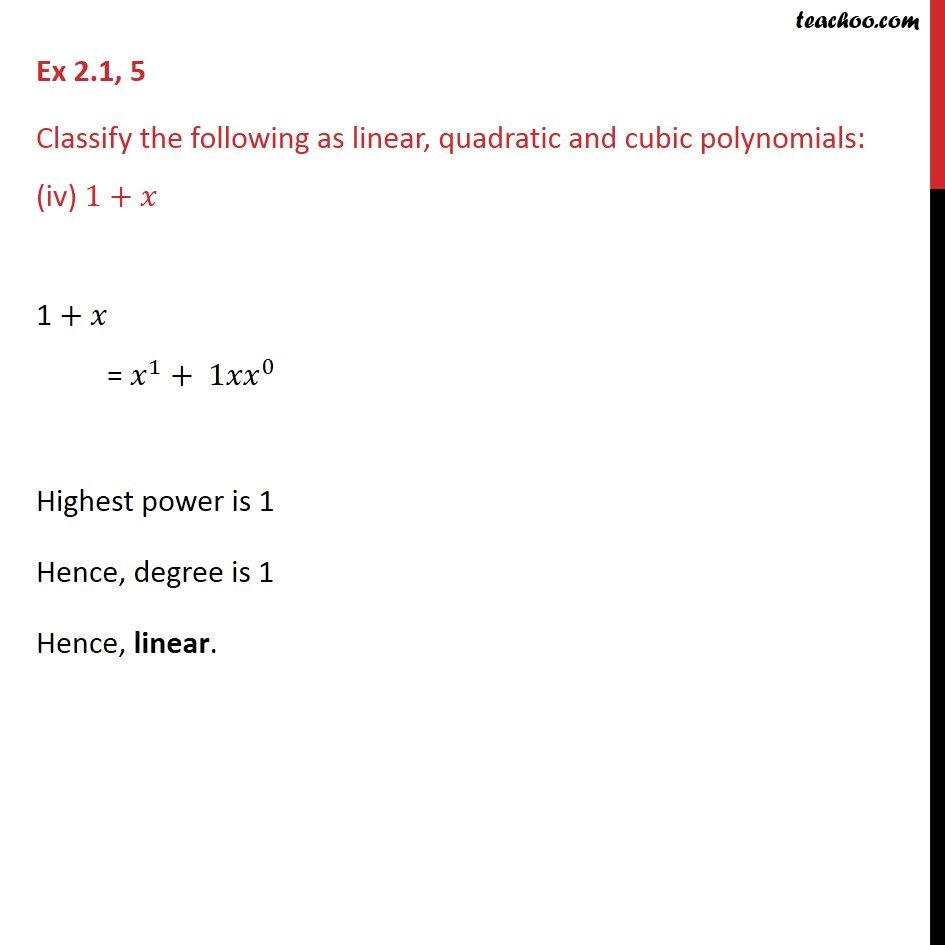1. Chapter 2 Class 9 Polynomials (Term 2)
2. Serial order wise
3. Ex 2.1

Transcript

Ex 2.1, 5 Classify the following as linear, quadratic and cubic polynomials: (iv) 1+ 1 + = 1 + 1 0 Highest power is 1 Hence, degree is 1 Hence, linear.